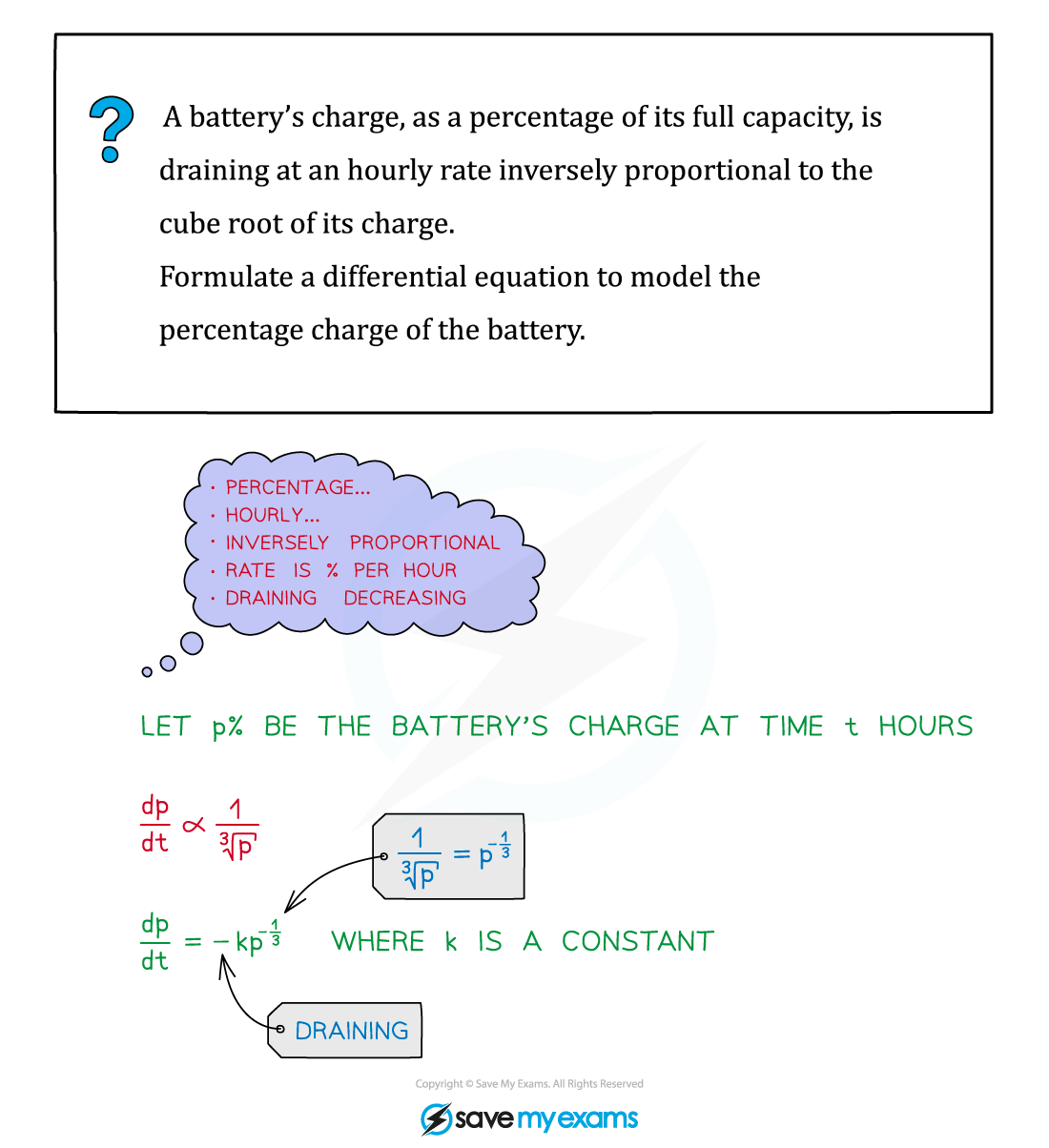# CIE A Level Maths: Pure 3复习笔记5.2.4 Modelling with Differential Equations

### Modelling with Differential Equations

#### What can be modelled with differential equations?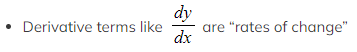There are many situations that involve “change”

• Temperature
• Medication
• Sales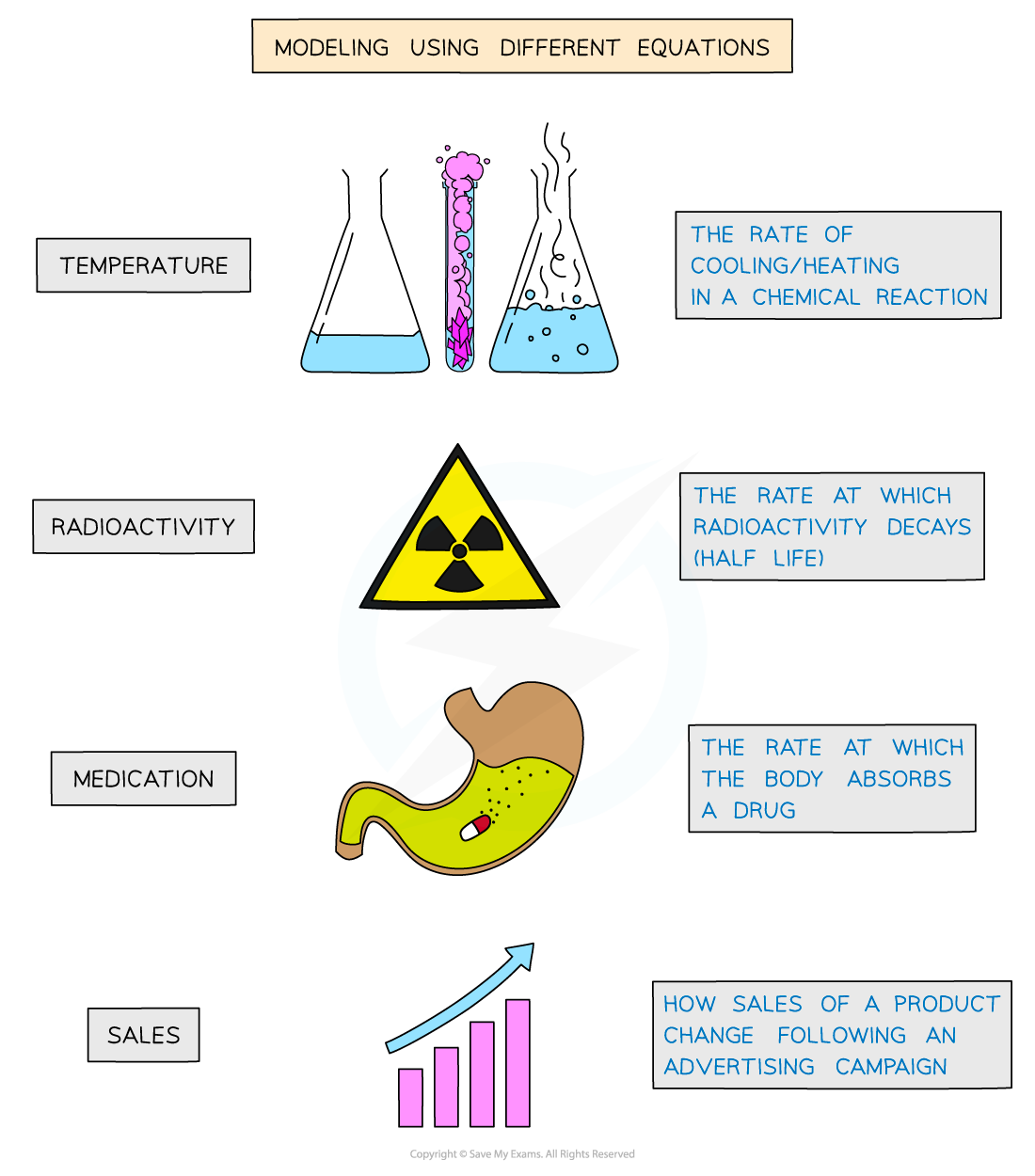#### How do I set up a model with differential equations?

• The first task is to set up a differential equation from a description in words: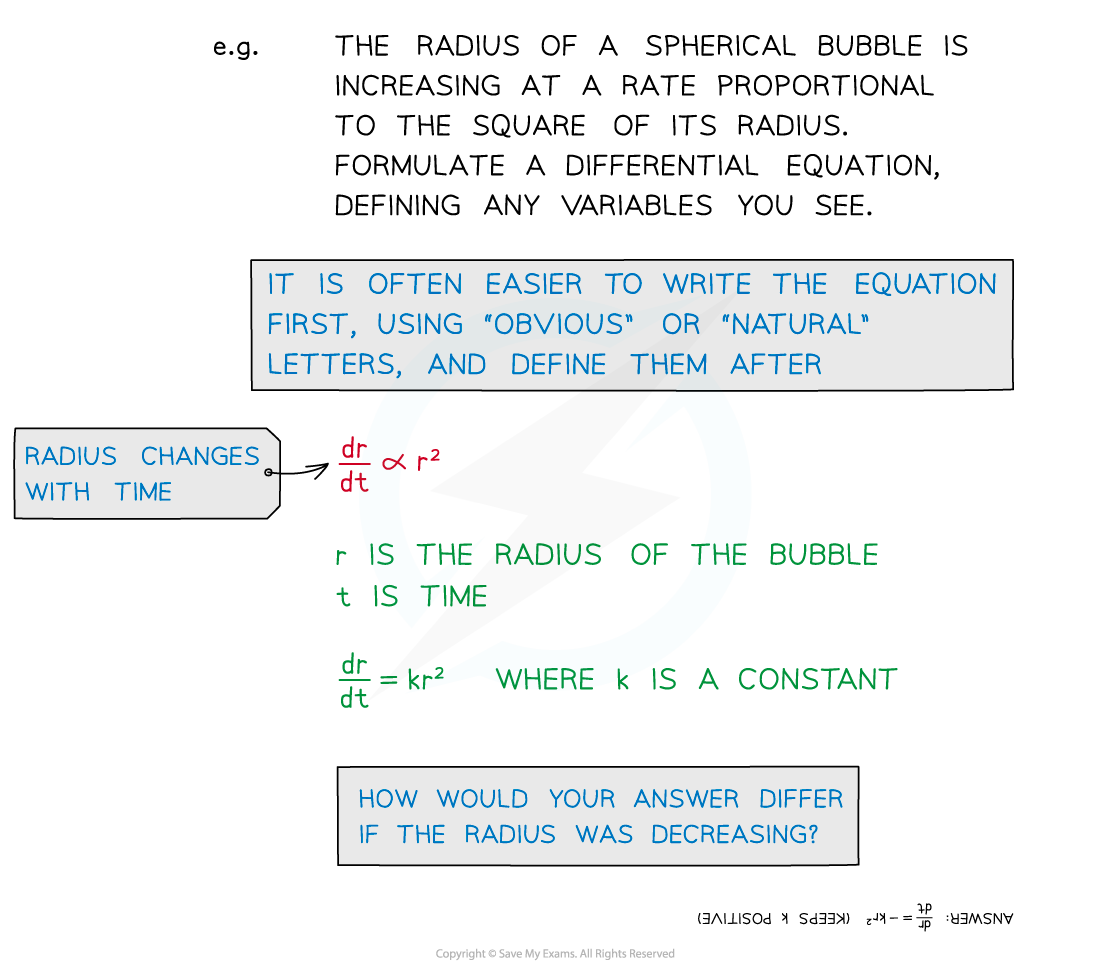• Important phrases here are …
•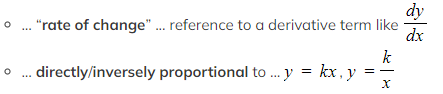… formulate ... means to write as an equation
• you may need to choose and define letters for variables
• V for volume, h for height (of a cylinder, say)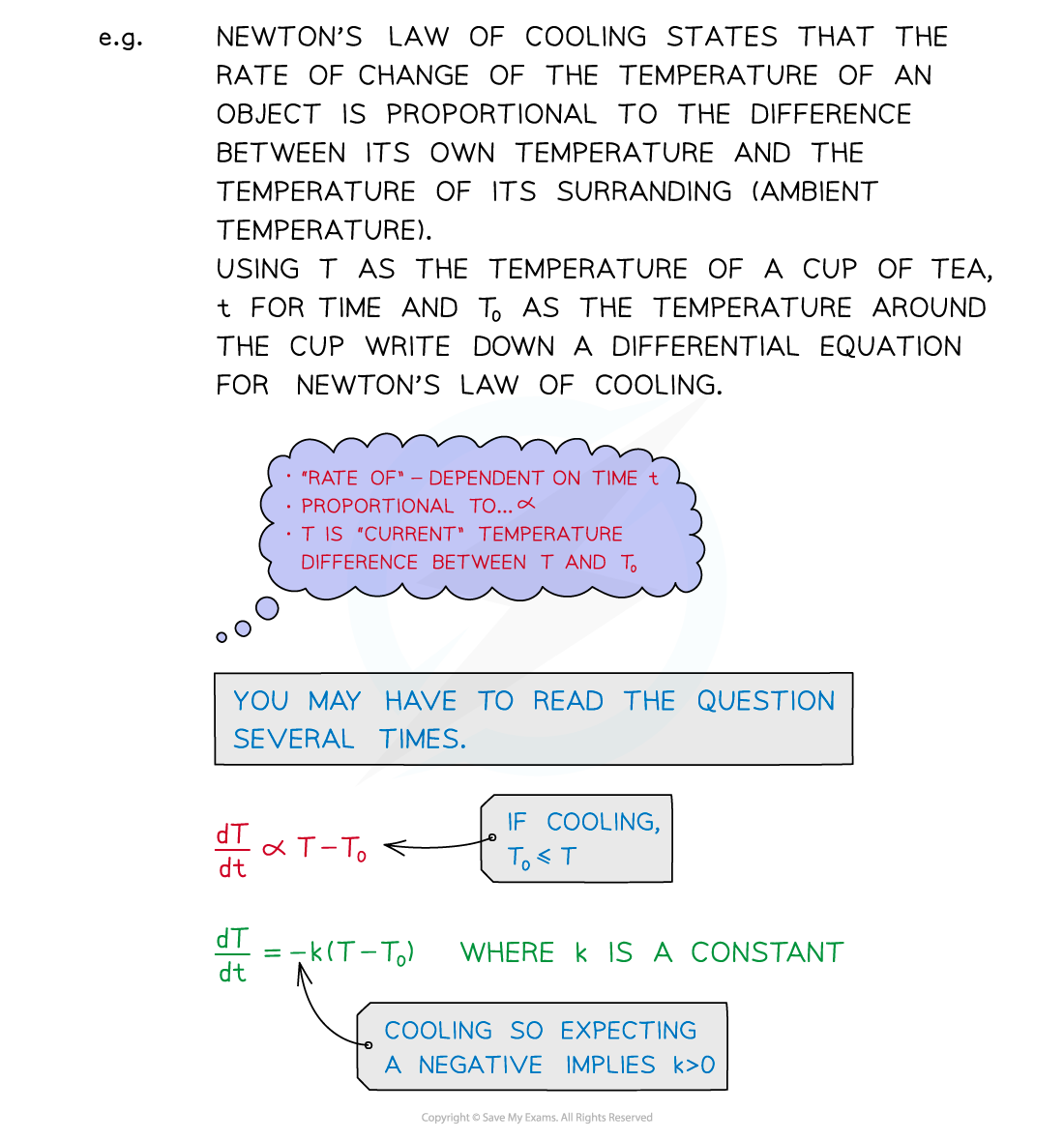• Some differential equations may involve Connected Rates of Change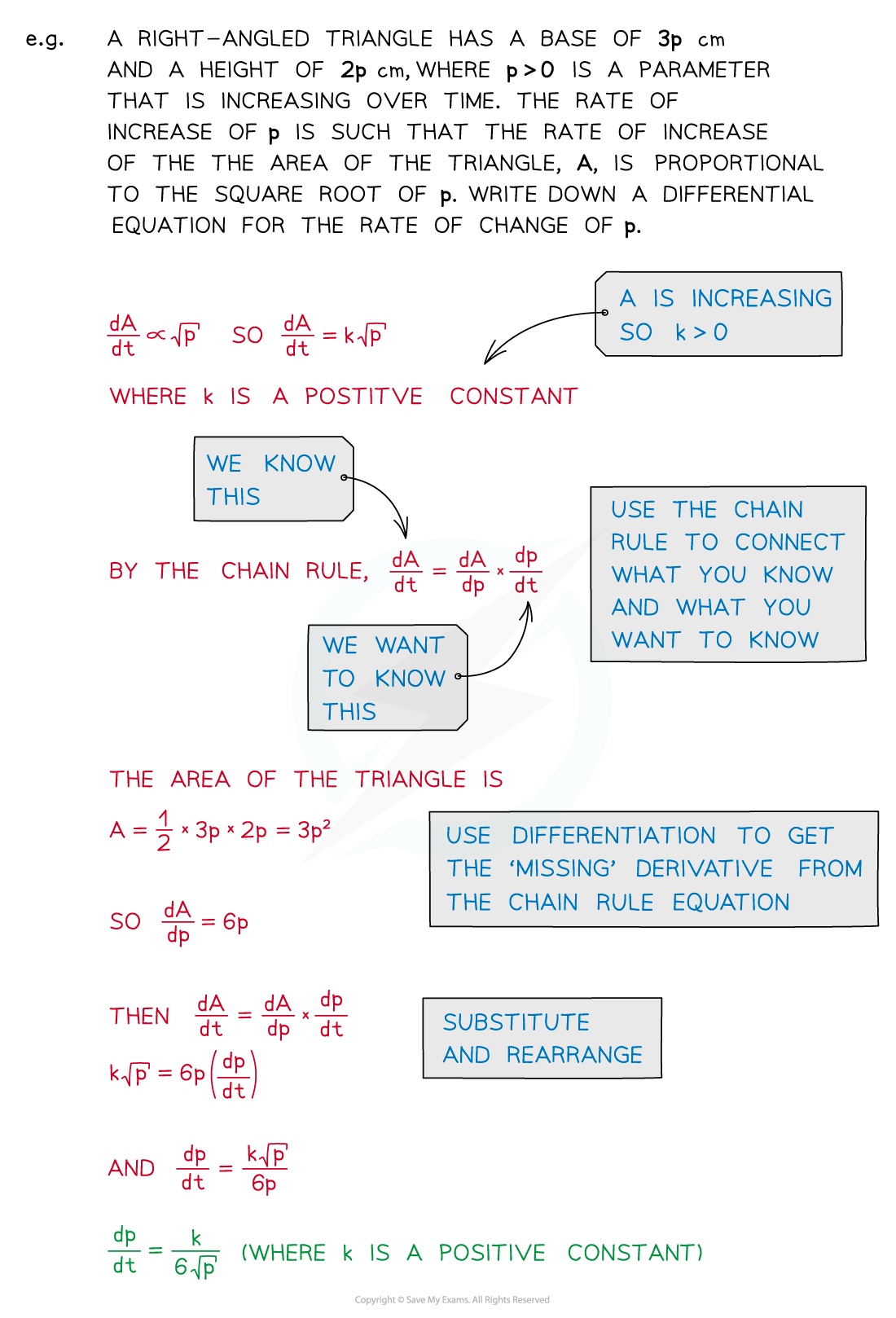#### Exam Tip

• Use a highlighter (or underline) to pick out important words/phrases
• Read and re-read the question several times
• Jot down bits and pieces as you go; do not expect to go straight from reading to writing down a differential equation.

#### Worked Example